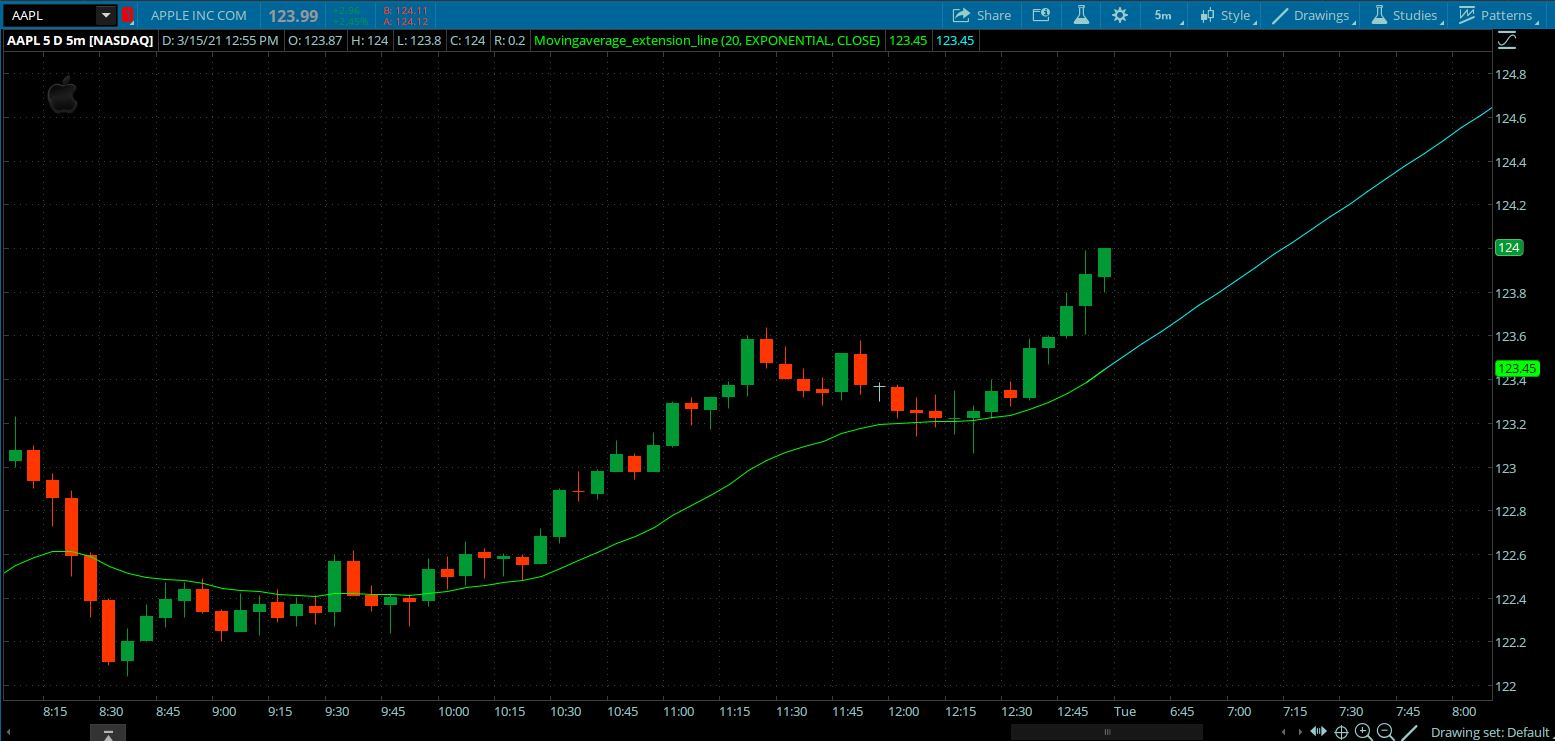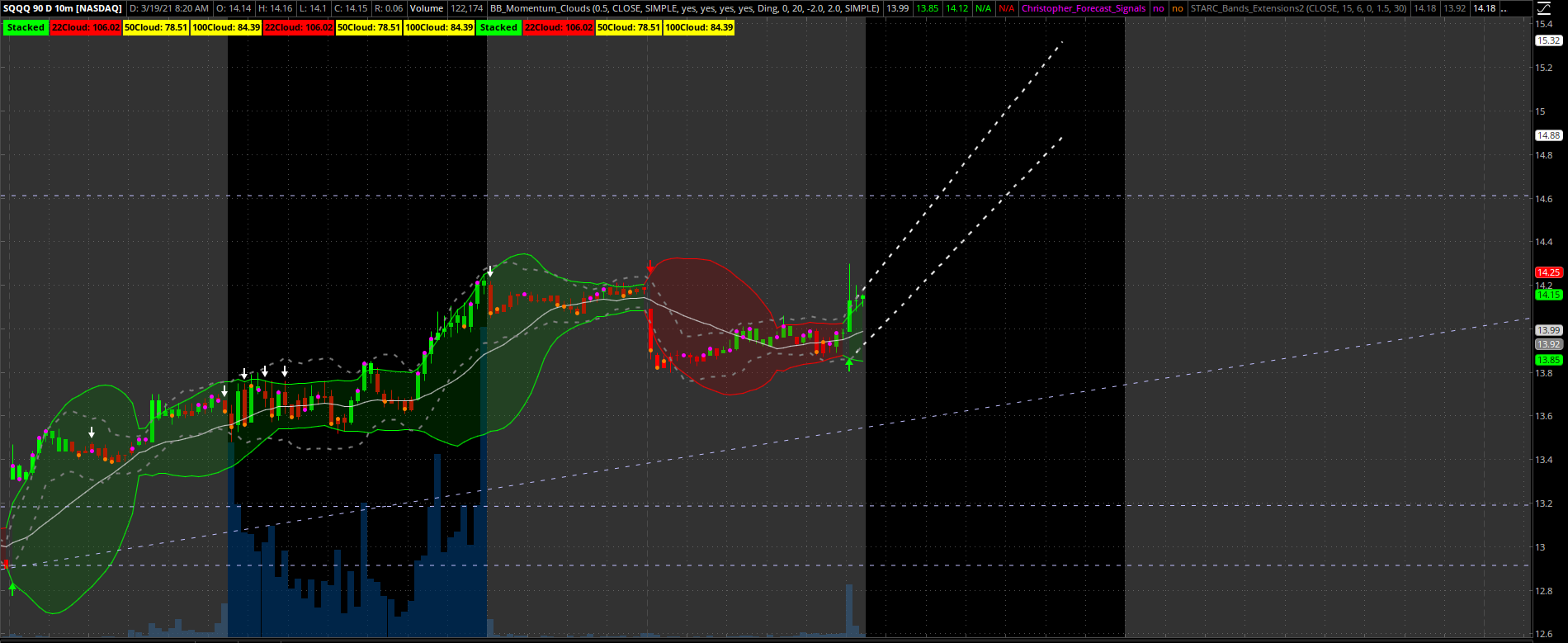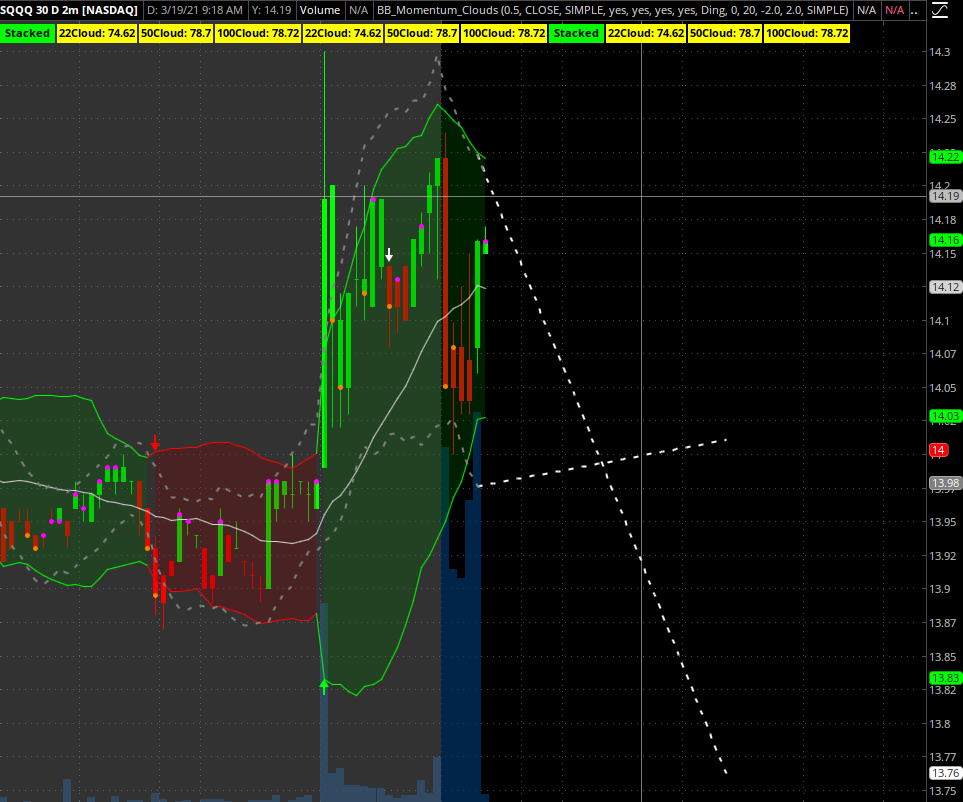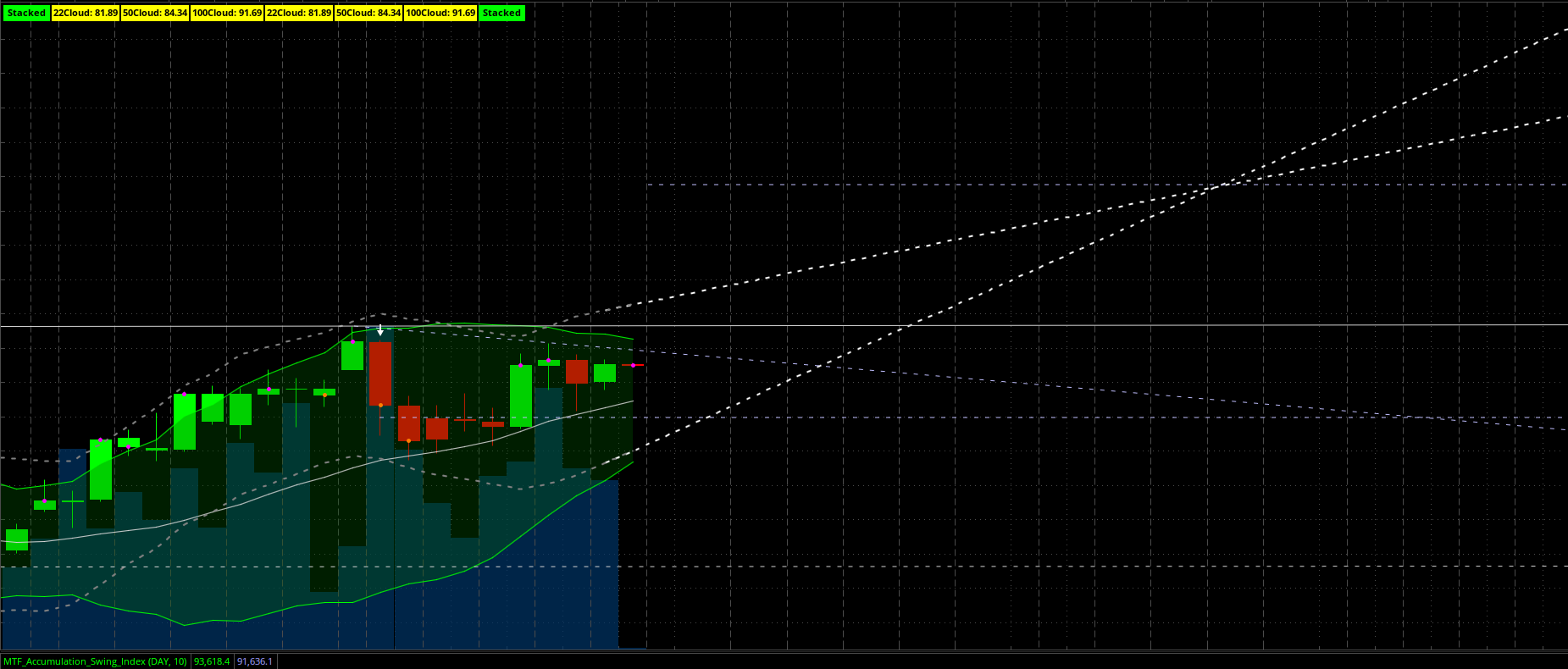# SMA extension

#### Christopher84

##### Well-known member
VIP
I know this is an odd question, but is it possible to extend the SMA 20 bars out? If so, how? Here is the SMA script. Any help is appreciated.

Code:
``````input price = close;
input length = 9;
input displace = 0;
input showBreakoutSignals = no;

plot SMA = Average(price[-displace], length);``````

Solution
Code:
``````declare weak_volume_dependency;

input price = close;
input ATR_length = 15;
input SMA_length = 6;
input displace = 0;
input multiplier_factor = 1.5;

def val = Average(price, sma_length);

def average_true_range = Average(TrueRange(high, close, low), length = atr_length);

plot Upper_Band = val[-displace] + multiplier_factor * average_true_range[-displace];
Upper_Band.SetStyle(Curve.SHORT_DASH);
Upper_Band.SetLineWeight(2);
Upper_Band.SetDefaultColor(Color.Gray);

def Middle_Band = val[-displace];
#Middle_Band.SetDefaultColor(GetColor(1));

plot Lower_Band = val[-displace] - multiplier_factor * average_true_range[-displace];
Lower_Band.SetStyle(Curve.SHORT_DASH);
Lower_Band.SetLineWeight(2)...``````
is it possible to extend the SMA 20 bars out?

What do you mean by that?

What do you mean by that?
Similar to the line extensions available when using the drawing feature except on sma instead of a static line. I was hoping to extend the line 20 bars forward.

Similar to the line extensions available when using the drawing feature except on sma instead of a static line. I was hoping to extend the line 20 bars forward.
Well sma, means simple moving average, you can't extend something that doesn't exist yet, meaning there's nothing to average yet, however you can push the present moving average onto the future

•BenTen
Well sma, means simple moving average, you can't extend something that doesn't exist yet, meaning there's nothing to average yet, however you can push the present moving average onto the future
I understand what sma means. I do not want to displace it into the future. I want to extend the end of it using its current slope.

perhaps a screenshot to accompany your explanation may possibly help alot

I understand what sma means. I do not want to displace it into the future. I want to extend the end of it using its current slope.

Look at the Ichimoku Study if you'd like to learn about extending forward... A site search should lead you there...

@Christopher84 You probably need to brute force the code with slope or delta and have each extension as a separate plot but there might be a better way.

I know this is an odd question, but is it possible to extend the SMA 20 bars out? If so, how? Here is the SMA script. Any help is appreciated.

Code:
``````input price = close;
input length = 9;
input displace = 0;
input showBreakoutSignals = no;

plot SMA = Average(price[-displace], length);``````

Here is an example from Mobius
Code:
``````# Plotting MA slope into extension area
# Mobius
# Chat Room Request

input n = 10;
input AvgType = AverageType.SIMPLE;
input price = close;

def MA = if !IsNaN(close())
then MovingAverage(AvgType, price, n)
else MA + ((MA - MA[n]) / (n - 1));

plot data = MA;``````

•XeoNoX
I added on to Mobius's code, all credits to him, i incorporated inertia plus made it so it uses the more recent data.

Plotting MA slope into extension area (Modded) .. All original credits to MOBIUS
Modded by XeoNoX for more recent data and incorporation of inertia in v2021.1
Draws extended line to the right of the chart based off recent linear regression curve of the Moving Average

(Most likely used for attempting future moving average prediction)
Code:
``````# Plotting MA slope into extension area (Modded v2021.1)
# Mobius
# Chat Room Request
#Modded by XeoNoX for more recent data and incorporation of inertia in v2021.1
#draws extended line to the right of the chart based off recent linear regression curve of the Moving Average
input MAlength = 20;
input AvgType = AverageType.SIMPLE;
input price = close;
plot moveavg = MovingAverage(AvgType, price, MAlength);
moveavg.SetDefaultColor(CreateColor(0, 255, 0));
moveavg.SetLineWeight(1);
def inertline = inertiaall(moveavg,2);
def EXT_MA = if !IsNaN(close()) then inertline else EXT_MA + ((EXT_MA - EXT_MA) / (2 - 1));
plot extension = EXT_MA;
extension.SetDefaultColor(CreateColor(0, 255, 255));
extension.SetLineWeight(1);``````Last edited:
def inertline = inertiaall(moveavg,2); def EXT_MA = if !IsNaN(close()) then inertline else EXT_MA + ((EXT_MA - EXT_MA) / (2 - 1)); plot extension = EXT_MA; extension.SetDefaultColor(CreateColor(0, 255, 255)); extension.SetLineWeight(1);
That is exactly what I am looking for! Thank you so much for your help!!!

I added on to Mobius's code, all credits to him, i incorporated inertia plus made it so it uses the more recent data.

Plotting MA slope into extension area (Modded) .. All original credits to MOBIUS
Modded by XeoNoX for more recent data and incorporation of inertia in v2021.1
Draws extended line to the right of the chart based off recent linear regression curve of the Moving Average

(Most likely used for attempting future moving average prediction)
Code:
``````# Plotting MA slope into extension area (Modded v2021.1)
# Mobius
# Chat Room Request
#Modded by XeoNoX for more recent data and incorporation of inertia in v2021.1
#draws extended line to the right of the chart based off recent linear regression curve of the Moving Average
input MAlength = 20;
input AvgType = AverageType.SIMPLE;
input price = close;
plot moveavg = MovingAverage(AvgType, price, MAlength);
moveavg.SetDefaultColor(CreateColor(0, 255, 0));
moveavg.SetLineWeight(1);
def inertline = inertiaall(moveavg,2);
def EXT_MA = if !IsNaN(close()) then inertline else EXT_MA + ((EXT_MA - EXT_MA) / (2 - 1));
plot extension = EXT_MA;
extension.SetDefaultColor(CreateColor(0, 255, 255));
extension.SetLineWeight(1);``````If I wanted to limit the extension length to 20 bars, what would be the correct way to code that?

If I wanted to limit the extension length to 20 bars, what would be the correct way to code that?

See if this helps
Code:
``````# Plotting MA slope into extension area (Modded v2021.1)
# Mobius
# Chat Room Request
#Modded by XeoNoX for more recent data and incorporation of inertia in v2021.1
#draws extended line to the right of the chart based off recent linear regression curve of the Moving Average
#Mod by Sleepyz to limit length of extension
input MAlength = 20;
input AvgType = AverageType.SIMPLE;
input price = close;
plot moveavg = MovingAverage(AvgType, price, MAlength);
moveavg.SetDefaultColor(CreateColor(0, 255, 0));
moveavg.SetLineWeight(1);
def inertline = inertiaall(moveavg,2);
def EXT_MA = if !IsNaN(close()) then inertline else EXT_MA + ((EXT_MA - EXT_MA) / (2 - 1));

input extension_length_limited_to = 20;
def lastbar = if isnan(close[-1]) and !isnan(close) then barnumber() else double.nan;

plot extension = if barnumber()<=highestall(lastbar)+ extension_length_limited_to then EXT_MA else double.nan;
extension.SetDefaultColor(CreateColor(0, 255, 255));
extension.SetLineWeight(1);``````

•XeoNoX
BTW @Christopher84 in your original post you said it was a odd question, i just would like to let you know your question really wasnt odd at all, i find this possibly being very useful. To be honest with you ive drawn them manually in the past if i needed to.

If you want the extension's value (possibly as a price projection) as a label just add this code to SleepyZ's code above in post #13
Code:
``AddLabel (yes, "Extension End: " +  round(highestall(extension),2)  );``

Last edited:
See if this helps
Code:
``````# Plotting MA slope into extension area (Modded v2021.1)
# Mobius
# Chat Room Request
#Modded by XeoNoX for more recent data and incorporation of inertia in v2021.1
#draws extended line to the right of the chart based off recent linear regression curve of the Moving Average
#Mod by Sleepyz to limit length of extension
input MAlength = 20;
input AvgType = AverageType.SIMPLE;
input price = close;
plot moveavg = MovingAverage(AvgType, price, MAlength);
moveavg.SetDefaultColor(CreateColor(0, 255, 0));
moveavg.SetLineWeight(1);
def inertline = inertiaall(moveavg,2);
def EXT_MA = if !IsNaN(close()) then inertline else EXT_MA + ((EXT_MA - EXT_MA) / (2 - 1));

input extension_length_limited_to = 20;
def lastbar = if isnan(close[-1]) and !isnan(close) then barnumber() else double.nan;

plot extension = if barnumber()<=highestall(lastbar)+ extension_length_limited_to then EXT_MA else double.nan;
extension.SetDefaultColor(CreateColor(0, 255, 255));
extension.SetLineWeight(1);``````
That did the trick! Thank you for sharing. I modified the code to work on the STARC Bands. It looks a bit like headlights on a car. It helps to show volatility and directionality (including reversals). I really appreciate the help.

•chinks1980, SleepyZ and XeoNoX
BTW @Christopher84 in your original post you said it was a odd question, i just would like to let you know your question really wasnt odd at all, i find this possibly being very useful. To be honest with you ive drawn them manually in the past if i needed to.

If you want the extension's value (possibly as a price projection) as a label just add this code to SleepyZ's code above in post #13
Code:
``AddLabel (yes, "Extension End: " +  round(highestall(extension),2)  );``
I’m glad to know I’m not the only one trying to draw them in. lol Would it be possible to have a point plot on the chart where the two extensions are crossed?

I’m glad to know I’m not the only one trying to draw them in. lol Would it be possible to have a point plot on the chart where the two extensions are crossed?
youll have to post a screenshot of what you mean. and what do you mean by point? a arrow?

youll have to post a screenshot of what you mean. and what do you mean by point? a arrow?
I think that the crossing points of the STARC Band extensions may show support and resistance zones. I’m wanting points or maybe dashes to show the zones. When I get to the office this morning, I’ll post the code if you think it’s a possibility.

Code:
``````declare weak_volume_dependency;

input price = close;
input ATR_length = 15;
input SMA_length = 6;
input displace = 0;
input multiplier_factor = 1.5;

def val = Average(price, sma_length);

def average_true_range = Average(TrueRange(high, close, low), length = atr_length);

plot Upper_Band = val[-displace] + multiplier_factor * average_true_range[-displace];
Upper_Band.SetStyle(Curve.SHORT_DASH);
Upper_Band.SetLineWeight(2);
Upper_Band.SetDefaultColor(Color.Gray);

def Middle_Band = val[-displace];
#Middle_Band.SetDefaultColor(GetColor(1));

plot Lower_Band = val[-displace] - multiplier_factor * average_true_range[-displace];
Lower_Band.SetStyle(Curve.SHORT_DASH);
Lower_Band.SetLineWeight(2);
Lower_Band.SetDefaultColor(Color.Gray);

#Plotting STARC slope into extension area
#input Extension_length = 20
input extension_length_limited_to = 50;
def lastbar = if isnan(close[-1]) and !isnan(close) then barnumber() else double.nan;
def inertline = inertiaall(Upper_Band,2);
def EXT_Upper_Band = if !IsNaN(close()) then inertline else EXT_Upper_Band + ((EXT_Upper_Band - EXT_Upper_Band) / (2 - 1));
plot extension = if barnumber()<=highestall(lastbar)+ extension_length_limited_to then EXT_Upper_Band else double.nan;
extension.SetStyle(Curve.SHORT_DASH);
extension.SetLineWeight(2);
extension.SetDefaultColor(Color.white);

def inertline2 = inertiaall(Lower_Band,2);
def EXT_Lower_Band = if !IsNaN(close()) then inertline2 else EXT_Lower_Band + ((EXT_Lower_Band - EXT_Lower_Band) / (2 - 1));
plot extension2 = if barnumber()<=highestall(lastbar)+ extension_length_limited_to then EXT_Lower_Band else double.nan;
extension2.SetStyle(Curve.SHORT_DASH);
extension2.SetLineWeight(2);
extension2.SetDefaultColor(Color.white);``````

declare weak_volume_dependency;

input price = close;
input ATR_length = 15;
input SMA_length = 6;
input displace = 0;
input multiplier_factor = 1.5;

def val = Average(price, sma_length);

def average_true_range = Average(TrueRange(high, close, low), length = atr_length);

plot Upper_Band = val[-displace] + multiplier_factor * average_true_range[-displace];
Upper_Band.SetStyle(Curve.SHORT_DASH);
Upper_Band.SetLineWeight(2);
Upper_Band.SetDefaultColor(Color.Gray);

def Middle_Band = val[-displace];
#Middle_Band.SetDefaultColor(GetColor(1));

plot Lower_Band = val[-displace] - multiplier_factor * average_true_range[-displace];
Lower_Band.SetStyle(Curve.SHORT_DASH);
Lower_Band.SetLineWeight(2);
Lower_Band.SetDefaultColor(Color.Gray);

#Plotting STARC slope into extension area
#input Extension_length = 20
input extension_length_limited_to = 50;
def lastbar = if isnan(close[-1]) and !isnan(close) then barnumber() else double.nan;
def inertline = inertiaall(Upper_Band,2);
def EXT_Upper_Band = if !IsNaN(close()) then inertline else EXT_Upper_Band + ((EXT_Upper_Band - EXT_Upper_Band) / (2 - 1));
plot extension = if barnumber()<=highestall(lastbar)+ extension_length_limited_to then EXT_Upper_Band else double.nan;
extension.SetStyle(Curve.SHORT_DASH);
extension.SetLineWeight(2);
extension.SetDefaultColor(Color.white);

def inertline2 = inertiaall(Lower_Band,2);
def EXT_Lower_Band = if !IsNaN(close()) then inertline2 else EXT_Lower_Band + ((EXT_Lower_Band - EXT_Lower_Band) / (2 - 1));
plot extension2 = if barnumber()<=highestall(lastbar)+ extension_length_limited_to then EXT_Lower_Band else double.nan;
extension2.SetStyle(Curve.SHORT_DASH);
extension2.SetLineWeight(2);
extension2.SetDefaultColor(Color.white);Last edited:
•JerryA

### Not the exact question you're looking for?

87k+ Posts
251 Online## The Market Trading Game Changer

Join 2,500+ subscribers inside the useThinkScript VIP Membership Club
• Exclusive indicators
• Proven strategies & setups
• Private Discord community
• Exclusive members-only content
• 1 full year of unlimited support

What is useThinkScript?

useThinkScript is the #1 community of stock market investors using indicators and other tools to power their trading strategies. Traders of all skill levels use our forums to learn about scripting and indicators, help each other, and discover new ways to gain an edge in the markets.

How do I get started?

We get it. Our forum can be intimidating, if not overwhelming. With thousands of topics, tens of thousands of posts, our community has created an incredibly deep knowledge base for stock traders. No one can ever exhaust every resource provided on our site.

If you are new, or just looking for guidance, here are some helpful links to get you started.

What are the benefits of VIP Membership?